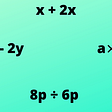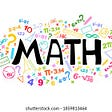# Bernoulli trial

What can we do to calculate probability of an event from a pair of independent event given the pair of event can occur in any possible order in a finite number of trials

As our events are binary we can attribute them as success and failure

Let’s say we want to calculate probability in n number of trials for k number of success given p is the probability of success

The formula is: nCk * P^n * (1-p)^n

Understanding the formula

1-P: probability of failure

n-k: failure count

What is the meaning and use of nCk in this formula?

It is the combination of all the possible ways k success and (n-k) failure can happen in n number of trials

Combination means permutation without order

Permutation nPk: n!/(n-k)!

For example if we want to arrange red, blue, green & black balls in pairs we will do 4*3 for permutation without repetition otherwise 4*4

It can be also written is 4!/(4–2)! thus the formula of permutation

For combination we just divide the formula by the number of seats factorial k! to get rid of the order

nCk = n!/k!(n-k)!

Let’s understand what we learned by an example, we need to find the combination for 5 trials given we need to find exactly 3 head

so the maximum number of head is 6 or the maximum number of success

SSS,SSF…..

thus k = 3, it is our hand & n = 5

5!/3!(5–3)!

it it was 10 trials and 4 success

k = 4, n = 10

10!/ 4!(10–4)!

My one confusion was aren’t number of hands = the number of trials? no, it is equal to the max no. of success

--

--

--

## More from Arnav Zedian

Finding Magic.

Love podcasts or audiobooks? Learn on the go with our new app.Finding Magic.

## Operations on Polynomial Class 10th## The secret to mastering basic Math## Matrix Multiplication: